Next: About this document ... Up: lab_template Previous: lab_template

Subsections

# Rectangular Approximations to Integrals

## Introduction

The purpose of this lab is to acquaint you with some rectangular approximations to integrals.

## Rectangular Approximations

Integration, the second major theme of calculus, deals with areas, volumes, masses, and averages such as centers of mass and gyration. In lecture you have learned that the area under a curve between two points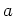and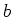can be found as a limit of a sum of areas of rectangles which approximate the area under the curve of interest. Not all area finding'' problems can be solved using analytical techniques. The Riemann sum definition of area under a curve gives rise to several numerical methods which can approximate the area of interest with great accuracy.

Suppose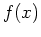is a non-negative, continuous function defined on some interval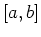. Then by the area under the curve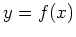between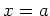andwe mean the area of the region bounded above by the graph of, below by the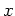-axis, on the left by the vertical line, and on the right by the vertical line. All of the numerical methods in this lab depend on subdividing the intervalinto subintervals of uniform length.

In these simple rectangular approximation methods, the area above each subinterval is approximated by the area of a rectangle, with the height of the rectangle being chosen according to some rule. In particular, we will consider the left, right and midpoint rules.

The Maple student package has commands for visualizing these three rectangular area approximations. To use them, you first must load the package via the with command. Then try the three commands given below to help you understand the differences between the three different rectangular approximations. Note that the different rules choose rectangles which in each case will either underestimate or overestimate the area.

> with(student):
> rightbox(x^2,x=0..4,3);
> leftbox(x^2,x=0..4,16);
> middlebox(x^2,x=0..4,10);

There are also Maple commands leftsum, rightsum, and middlesum to sum the areas of the rectangles, see the examples below. Note the use of evalf to obtain the desired numerical answers.
> rightsum(x^2,x=0..4);
> evalf(rightsum(x^2,x=0..4,3));
> evalf(leftsum(x^2,x=0..4,16));
> evalf(middlesum(x^2,x=0..4,10));


## Accuracy

It should be clear from the graphs that adding up the areas of the rectangles only approximates the area under the curve. However, by increasing the number of subintervals the accuracy of the approximation can be improved. One way to measure how good the approximation is is the absolute error, which is the difference between the actual answer and the estimated answer. Later on in the course, you will learn techniques for finding the exact answer. Approximations, however, are important because exact answers cannot always be found.

All of the Maple commands described so far in this lab can include a third argument to specify the number of subintervals. The default is 4 subintervals. The example below approximates the area under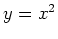from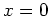to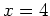using the rightsum command with 50, 100, 320 and 321 subintervals. As the number of subintervals increases, the approximation gets closer and closer to the exact answer. You can see this by assigning a label to the approximation, assigning a label to the exact answer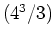and taking their difference. The closer you are to the actual answer, the smaller the difference. The example below shows how we can use Maple to approximate this area with an absolute error no greater than 0.1.

> exact := 4^3/3;
> estimate := evalf(rightsum(x^2,x=0..4,50));
> evalf(abs(exact-estimate));
> estimate := evalf(rightsum(x^2,x=0..4,100));
> evalf(abs(exact-estimate));
> estimate := evalf(rightsum(x^2,x=0..4,320));
> evalf(abs(exact-estimate));
> estimate := evalf(rightsum(x^2,x=0..4,321));
> evalf(abs(exact-estimate));


## Exercises

1. Plot the function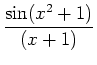on the intervalwith 6 rectangles determined by the right-endpoint rule. Then plot the function with 6 rectangles using the left-endpoint rule.
1. Which rule overestimates the area for the given function? Will this rule always overestimate the area for any function? Why?
2. Is the midpoint rule an average of the left and right endpoint rules? Verify your claim using the above function with 6 rectangles.

2. The triangle with vertices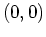,, and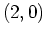has an exact area of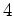.
1. What are the two linear equations that bound this triangle above and below? What is the interval ofvalues that bound this triangle? Supply a plot of these lines over this interval.
2. Use the command rightsum to find the minimum number of subintervals needed to approximate this area with error less than 0.1.

3. The exact value of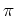can be defined by the definite integral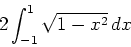since this integral represents the area of a circle of radius 1.

1. Use the command leftsum to find the minimum number of subintervals needed to approximate the value ofwith error no greater than 0.01.

2. Use the command middlesum to find the minimum number of subintervals needed to approximate the value ofwith error no greater than 0.01.

3. Explain which estimation you think is better and why.Next: About this document ... Up: lab_template Previous: lab_template
Dina Solitro
2007-01-14